Function Repository Resource:

# ZXDiagramToQuantumDiscreteState

Convert a ZX-diagram into a pure quantum discrete state

Contributed by: Jonathan Gorard
 ResourceFunction["ZXDiagramToQuantumDiscreteState"][ZXDiagramObject[…]] converts the specified ZXDiagramObject into an equivalent (pure) QuantumDiscreteState.

## Details

If ResourceFunction["ZXDiagramToQuantumDiscreteState"] succeeds in converting the specified ZXDiagramObject into a pure discrete quantum state, it will return a QuantumDiscreteState object.
ResourceFunction["ZXDiagramToQuantumDiscreteState"] works by converting each Z-spider generator in the specified ZXDiagramObject into a qubit of the general form {1,α}, and each X-spider generator in the specified ZXDiagramObject into a qubit of the general form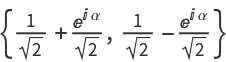, before taking a tensor product of all such qubits to yield the desired QuantumDiscreteState.
Since it is representing a (pure) QuantumDiscreteState, every generator/spider within the specified ZXDiagramObject should have an input arity of 0 and an output arity of 1.
In the resulting QuantumDiscreteState, the following properties are supported:
 "Amplitudes" association <|b1→amp1,b2→amp2,…|> of basis names and amplitudes "Basis" which QuantumBasis the state is defined with respect to "Picture" which quantum mechanical picture the state is defined with respect to "BasisElements" list of basis elements bi "StateVector" state vector {amp1,amp2,…} (for pure states only) "DensityMatrix" density matrix mat (for both pure and mxied states) "VonNeumannEntropy" Von Neumann entropy (entanglement entropy) of the state "Purity" purity of the quantum state, specified as a real number "PureStateQ" whether the state is pure (purity equals 1) "MixedStateQ" whether the state is mixed (purity is not equal to 1) "Qudits" number of qudits (subsystems) within the state "Dimensions" dimensionality of each qudit (subsystem) "BlochSphericalCoordinates" spherical coordinates of the state on the Bloch sphere (qubits only) "BlochCartesianCoordinates" Cartesian coordinates of the state on the Bloch sphere (qubits only) "Properties" list of all property names
The default convention is to name Z-spiders as z1,z2,; X-spiders as x1,x2,; black diamonds as d1,d2,; outputs as o1,o2,; etc.

## Examples

### Basic Examples (2)

Convert a single-spider ZX-diagram into a single-qubit pure discrete quantum state:

 In:=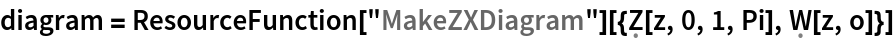Out=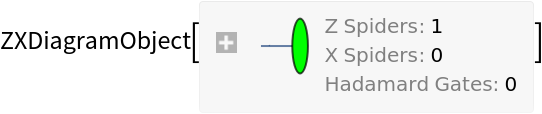In:=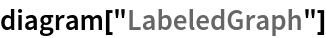Out=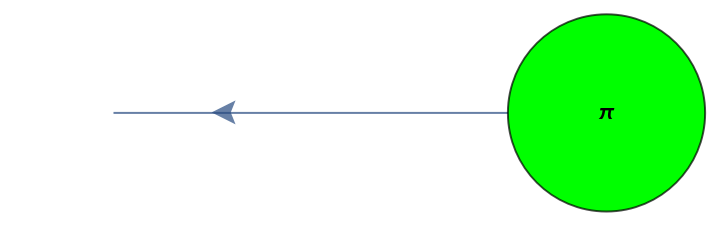In:=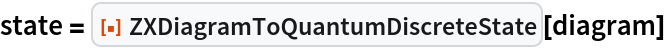Out=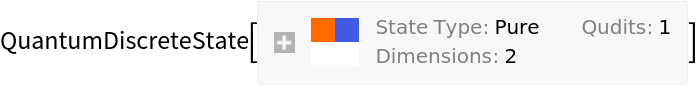In:=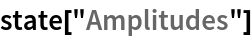Out=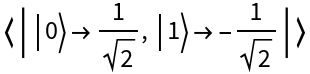Convert a different single-spider ZX-diagram into a single-qubit pure discrete quantum state:

 In:=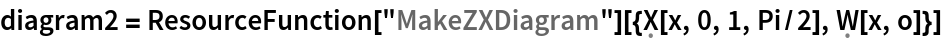Out=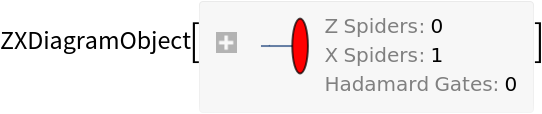In:=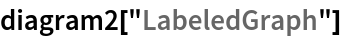Out=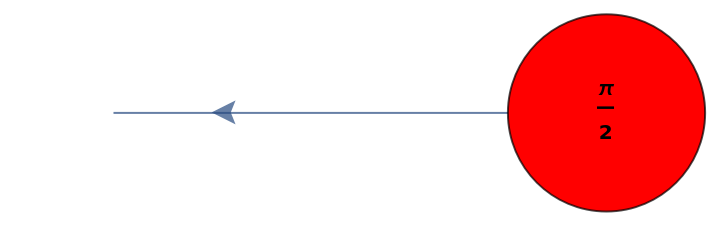In:=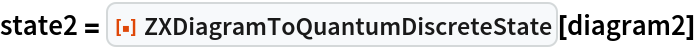Out=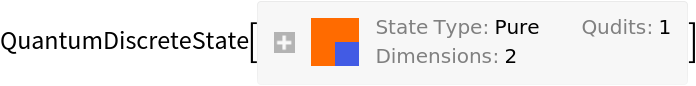In:=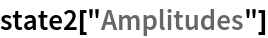Out=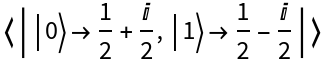Convert a more complicated three-spider ZX-diagram into a three-qubit pure discrete quantum state:

 In:=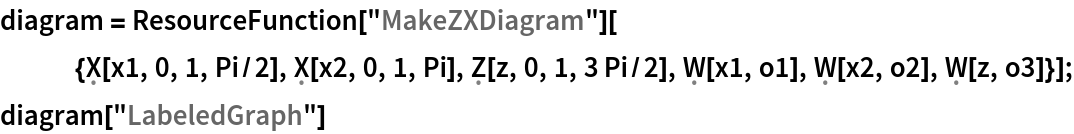Out=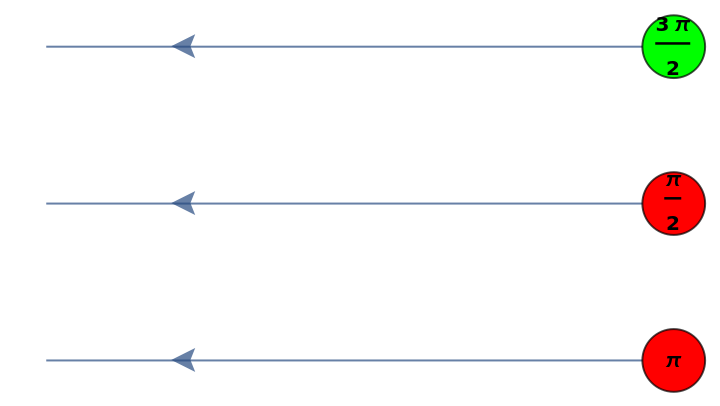In:=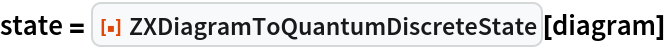Out=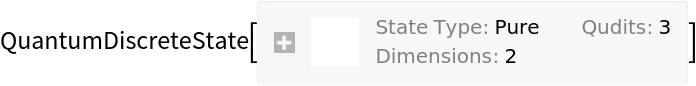In:=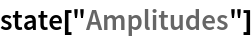Out=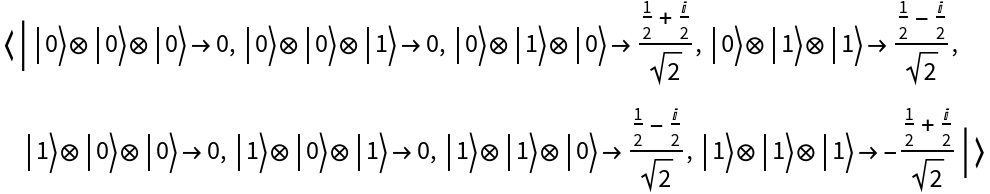Convert a different four-spider ZX-diagram into a four-qubit pure discrete quantum state:

 In:=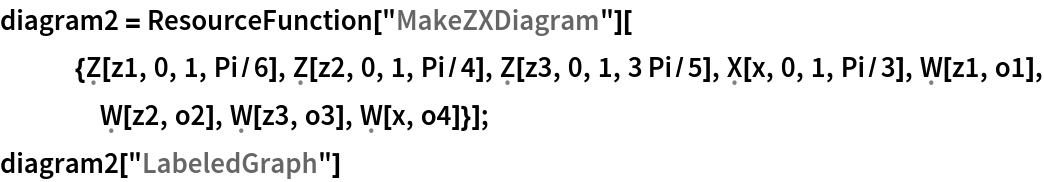Out=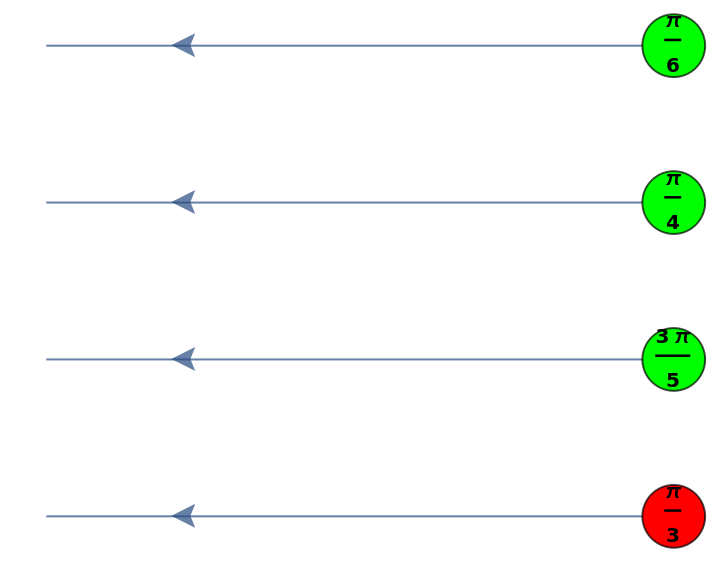In:=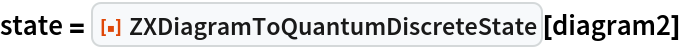Out=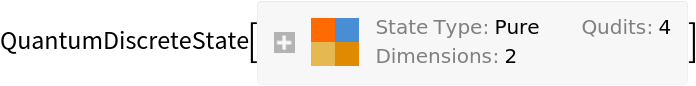In:=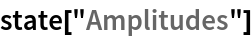Out=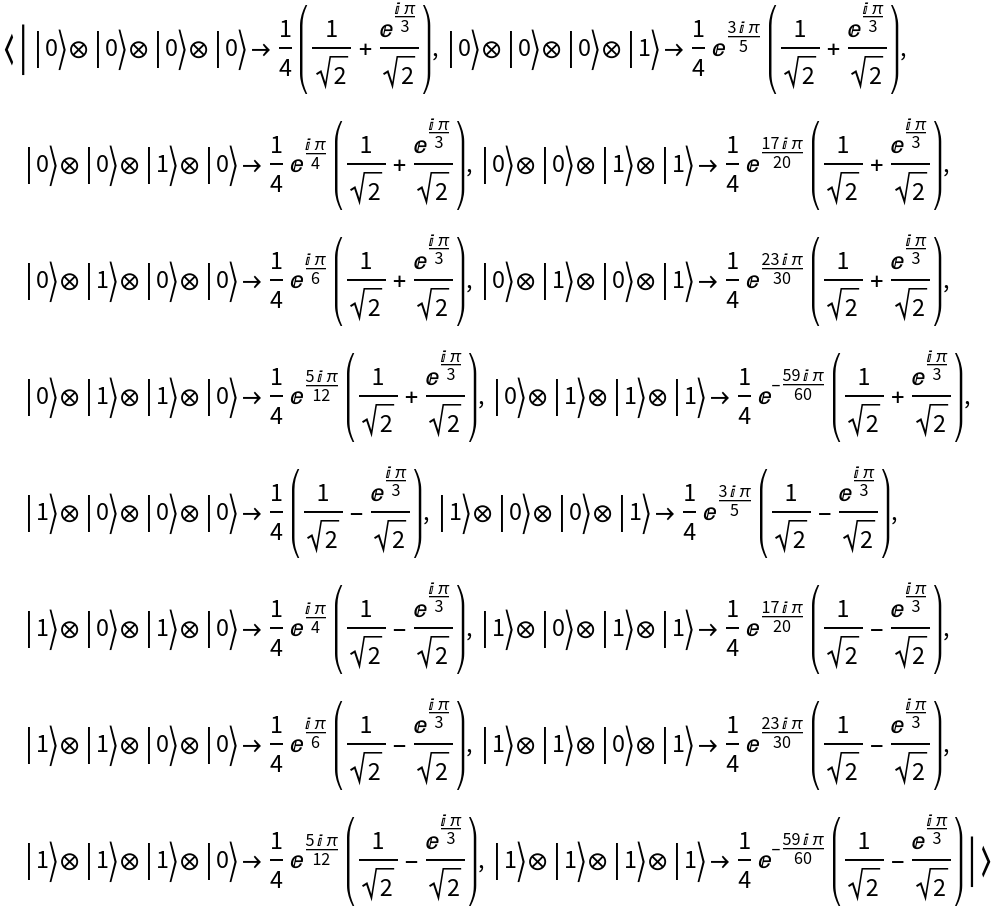Jonathan Gorard

## Version History

• 1.0.0 – 26 April 2021